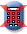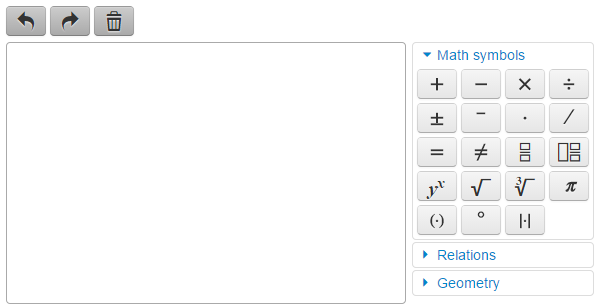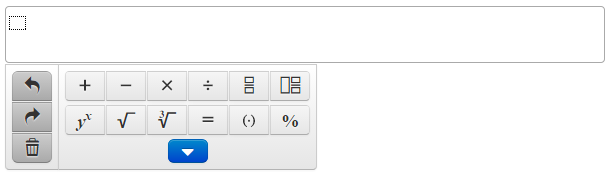Massachusetts Comprehensive Assessment SystemReporting Category: Expressions and Equations
Standard: CCSS.Math.Content.6.EE.C.9 - Use variables to represent two quantities in a real-world problem that change in relationship to one another; write an equation to express one quantity, thought of as the dependent variable, in terms of the other quantity, thought of as the independent variable. Analyze the relationship between the dependent and independent variables using graphs and tables, and relate these to the equation. For example, in a problem involving motion at constant speed, list and graph ordered pairs of distances and times, and write the equation d = 65t to represent the relationship between distance and time.
Item Description: Analyze the relationship between the variables in a table to create and solve an equation that represents a given real-world context.

This table shows the relationship between the number of pies sold at a farm and the total profit made, in dollars, from the pies sold at the farm.#### Part A

Based on the table, what is the profit made, in dollars, from each pie sold at the farm? Show or explain how you got your answer.#### Part B

Write an equation that can be used to find p, the total profit made, in dollars, when n pies are sold at the farm.#### Part C

Use the equation you wrote in Part B to calculate the profit made, in dollars, when 15 pies are sold at the farm. Show or explain how you got your answer.#### Part D

One day, the farm made a profit of \$144 from the pies sold. How many pies were sold at the farm on that day? Show or explain how you got your answer.## Scoring Guide and Sample Student Work

Select a score point in the table below to view the sample student response.

ScoreDescription
4 The student response demonstrates an exemplary understanding of the Expressions and Equations concepts involved in using variables to represent two quantities in a real-world problem that change in relationship to one another; and writing an equation to express one quantity, thought of as the dependent variable, in terms of the other quantity, thought of as the independent variable. The student writes an equation to represent the relationship between two quantities and uses the equation to calculate the value of one quantity, given the other.
4
3 The student response demonstrates a good understanding of the Expressions and Equations concepts involved in using variables to represent two quantities in a real-world problem that change in relationship to one another; and writing an equation to express one quantity, thought of as the dependent variable, in terms of the other quantity, thought of as the independent variable. Although there is significant evidence that the student was able to recognize and apply the concepts involved, some aspect of the response is flawed. As a result the response merits 3 points.
2 The student response demonstrates a fair understanding of the Expressions and Equations concepts involved in using variables to represent two quantities in a real-world problem that change in relationship to one another; and writing an equation to express one quantity, thought of as the dependent variable, in terms of the other quantity, thought of as the independent variable. While some aspects of the task are completed correctly, others are not. The mixed evidence provided by the student merits 2 points.
1 The student response demonstrates a minimal understanding of the Expressions and Equations concepts involved in using variables to represent two quantities in a real-world problem that change in relationship to one another; and writing an equation to express one quantity, thought of as the dependent variable, in terms of the other quantity, thought of as the independent variable.
0 The student response contains insufficient evidence of an understanding of the Expressions and Equations concepts involved in using variables to represent two quantities in a real-world problem that change in relationship to one another; and writing an equation to express one quantity, thought of as the dependent variable, in terms of the other quantity, thought of as the independent variable to merit any points.
Note: There are 2 sample student responses for Score Point 4.

Question 5: Expressions and Equations

Last Updated: July 1, 2020